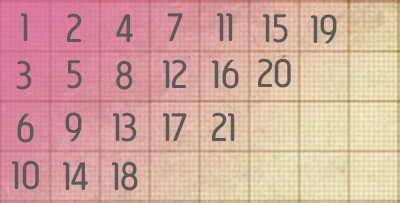# jQuery Diagonal Grid Fill Algorithm

This week, the team mulled over a compelling code challenge: “How do you fill a grid in diagonal columns starting at the top left corner?”

As a person who legitimately takes pleasure in solving trivial puzzles, I approached the problem head-on. And although easy to solve on paper, the evolution of a grid quickly became tricky to invent for web use. But alas, after a couple Redbulls I came to a solution.To achieve the diagonal grid fill algorithm, only the height and width of the grid are necessary. Each diagonal column must be filled from top to bottom, left to right. It’s achieved by a loop and decrements to the selected cell by (width-1) and (height-1). The loop continues until the selected cell no longer exists.

Below is a PHP representation of the algorithm.

```<?php
\$grid = array(array());
\$h = 4;
\$w = 5;
\$count = 1;
for (\$i = 1; \$i <= \$w+\$h; \$i++) {
\$index = min(\$i,\$w);
\$row = max(1,(\$i-(\$w+1)+2));
while (\$index != 0 && \$row <= \$h) {
\$grid[\$row][\$index] = \$count;
echo \$row.','.\$index.' = '.\$count.'<br />';
\$count++;
\$index--;
\$row++;
}
}
?>
```

From here, implementing the code into JavaScript was fairly easy. With only a few simple syntax changes and a new grid from divs, the desired product was created.

```<script type="text/javascript">
function vuurrPixel(arg) {
var size = 40;
var h = \$('.screen').height() / size;
var w = \$('.screen').width()  / size;
var count = 1;

for(i = 1; i <= (w + h); i++) {
var index = Math.min( i , w );
var row = Math.max(1,(i - ( w + 1 ) + 2 ) );

while (index != 0 && row <= h) {
if (arg == 'show') {
\$('.screen').append('<div style="display: none;" id="block-'+index+'-'+row+'" class="pixel"></div>');
\$('.block').animate({opacity:"show"}, 1500);
}else if (arg == 'hide') {
\$('.block').animate({opacity:"hide"}, 1500);
}
count++;
index--;
row++;
}
}
}
</script>
```

My problem conquered, I see many uses for it in the future. The diagonal grid fill algorithm can be used in many applications (slides, menus, etc…). Personally, my next feat is to use HTML5 canvases to implement the same algorithm for mobile compatibility.

## GitHub Gist